# Community

Blog Events Webinars Tutorials Forum
×
Community Blog Formal Verification Tool TLA+: An Introduction from the Perspective of a Programmer

# Formal Verification Tool TLA+: An Introduction from the Perspective of a Programmer

A programmer offers perspective and reintroduces TLA+ using his experiences.

## Preface

Theoretically, it is difficult to prove the correctness of a program or algorithm. Tests are generally used in engineering to discover problems, but no amount of tests can guarantee that all behaviors are covered. Those behaviors that are not covered become potential risks. Once exposed online, they will cause unexpected results. Formal verification can solve such problems. It can perform exhaustive searches for all possible behaviors, check whether the preset attributes are met, and find any behaviors that do not meet expectations through its powerful computing capabilities. It can fundamentally guarantee the correctness of the algorithm.

## An Introduction to TLA+

Temporal Logic of Actions (TLA+) is a formal verification language developed by Leslie Lamport. It is used for program designing, modeling, documentation, and verification, especially concurrent systems and distributed systems. TLA+ was designed to describe the system with simple mathematical theories and formulas. TLA+ and the related tools help eliminate basic errors that are difficult to discover in programs and costly to correct.

You can use TLA+ to perform formal verification of the program. First, you can use TLA+ to describe the program. This kind of description is called a specification. You can use the TLC model checker with a specification to run it. The running process will traverse all possible behaviors, check the attributes set in a specification, and find unexpected behaviors.

TLA+ is based on mathematics, and it adopts mathematical thinking. It is not similar to any programming language. Lamport developed the PlusCal language to lower the threshold of TLA+. PlusCal is similar to a programming language, which can describe the program logic and translate PlusCal into TLA+ by borrowing the tools provided by TLA+. Most engineers will find that PlusCal is the easiest way to start using TLA+, but the issue is that PlusCal does not have some functions of TLA+. Sometimes it cannot construct complex models like TLA+, so PlusCal cannot replace TLA+. First, you can use the PlusCal programming language to complete the basic logic and modify it further based on the generated TLA+ code, which can simplify the development of TLA+.

## TLA+ Application

TLA+ is widely used in academia and industrial circles. TLA+ Examples provide some distributed algorithms and concurrent algorithms that have been verified by TLA+. TLA+ verification is a basic standard for proposing a new algorithm from an existing algorithm in the research fields of distributed algorithms and concurrent algorithms. In addition to the introduction of non-formal argumentation, many distributed algorithm papers will attach a specification of TLA+ to prove that their algorithms are verified by formal verification. For people in the industry familiar with TLA+, reading the specification of TLA+ is even faster than reading the paper. When the language description of the paper is not clear, or when readers feel ambiguous, checking the Specification of TLA+ is a good tool for reading and understanding the paper. Sometimes some algorithm details can only be seen in the Specification of TLA+. It can be better used as a guide for implementation since the specification is logically rigorous.

Lamport's TLA+ homepage lists some of the TLA+ industry applications. The core algorithms of some Amazon AWS systems use TLA+ for formal verification. For example, Table 1 lists the problems found by TLA+ for some AWS systems, which covers core components. Once the problems of these core components are exposed online, the losses will be immeasurable. Just like this, it has become an industry standard for the core algorithm of distributed cloud services to use TLA+ to carry out design verifications. Therefore, as cloud service practitioners or learners interested in this, being familiar with TLA+ is an indispensable bonus.

Table 1: Problems Identified by TLA+ for AWS Systems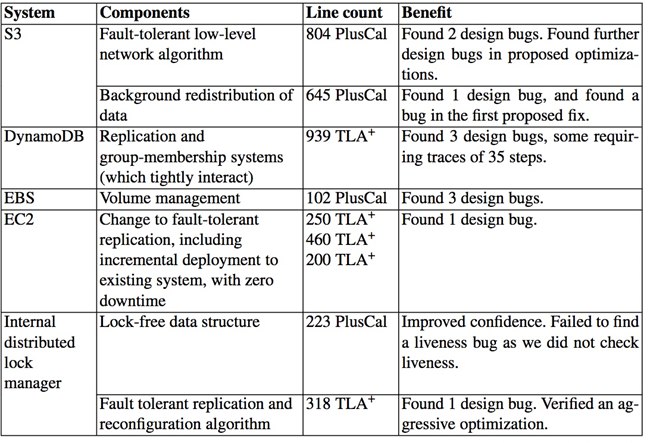## Getting Started with TLA+

You can install the TLA+ plug-in in VS Code to start using TLA+. Let's start with a simple example:

You can think of a single-bit clock. Since there is only one bit, it can only take the value 0 or 1. Its behavior is in the following two cases:

0 -> 1 -> 0 -> 1 -> 0 -> ...
1 -> 0 -> 1 -> 0 -> 1 -> ...

How do you use TLA+ to describe the clock? You can use PlusCal to get started, which is more convenient for engineers:

-------------------------- MODULE clock----------------------
(*--fair algorithm Clock          \*PlusCal code is written in TLA+ comments
variable
clock \in {0, 1};             \*define the variable and initialize it to 0 or 1
define
Inv == clock \in {0, 1}       \*Define the invariant
end define
begin
while TRUE do                 \*infinite loop
if clock = 0 then         \*if the value of clock is 0
clock := 1;           \*change the value of clock to 1
else                      \*otherwise
clock := 0;           \*change the value of clock to 0
end if
end while
end algorithm;*)                  \*end algorithm
============================================================

Figure 1: PlusCal Description of a Single-Bit Clock

Figure 1 is a PlusCal description of a single-bit clock, which can be understood easily by learners with basic programming skills. This PlusCal code can be translated into TLA+ code directly using the tools provided by TLA+:

------------------------ MODULE clock------------------------
VARIABLE clock                    \*declare clock variables
vars == << clock >>               \*declare variable list
Inv == clock \in {0, 1}           \*define invariant
Init == clock \in {0, 1}          \*initialization
Tick ==                           \*clock Tick
IF clock = 0              \*If the value of clock is 0
THEN clock' = 1        \*change the value of clock to 1
ELSE clock' = 0        \* change the value of clock to 0
Spec ==                           \*Specification
/\ Init                   \*Initialize, and /\is the logical and
/\ [][Tick]_vars          \*Tick is always true or keeps variables unchanged
/\ WF_vars(Tick)          \*prevent Tick from never executing
============================================================

Figure 2: TLA+ Description of a Single-Bit Clock

With the basis of the preceding PlusCal, it is not difficult to understand this section of TLA+. The focus is on the understanding of Spec. Spec defines the behavior of the system. Figure 3 describes the behavior of a single-bit clock. Init initializes the clock to 0 or 1, Tick makes the clock jump back and forth between 0 and 1, and Stutter makes the clock remain unchanged. The process of TLA+ running is traversing the figure.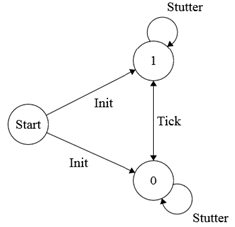Figure 3: Behavior of a Single-Bit Clock

The preceding TLA+ code needs to be saved to the clock.tla file to make this TLA+ run. In addition, a clock.cfg file needs to be written (as shown in Figure 4.) The content of the clock.cfg file is simple. It indicates which specification to run and which invariant to check.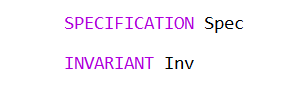Figure 4: Contents of the clock.cfg File

You can use TLC to run using these two files. Then, you will get the results shown in Figure 5, which shows some statistical information.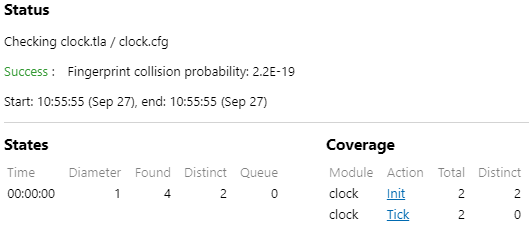Figure 5: Running Results

## TLA+ Principle

You can add some parameters during operation to understand the operation principle of TLA+ and find out how it traverses and make TLC output the status diagram. For example, we run a section of TLA+ code shown in Figure 6. Figure 7 is the cfg file needed to run. This example attempts to find all the combinations of 19 dollars with nominal values of 1, 2, and 5.

-------------------------- MODULE money  ------------------------
EXTENDS Integers                            \*introduce Integers modules
CONSTANT MONEYS                             \*declare MONEYS constants
CONSTANT TOTAL                              \*declare TOTAL constants
VARIABLES money                             \*declare money variables
Init == money = 0                           \*initialization
Add(i) == /\ money + i <= TOTAL             \*if not more than TOTAL
/\ money' = money + i             \*Add money
Terminating == /\ \A i \in MONEYS: money + i > TOTAL
/\ UNCHANGED <<money>>       \*Keep money unchanged
\/ Terminating                      \*or terminate
Inv == money <= TOTAL                       \*invariant
==================================================================

Figure 6: money.tla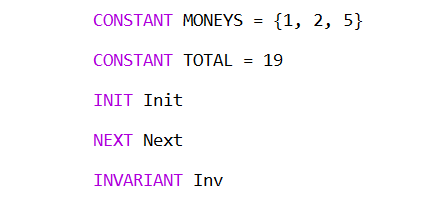Figure 7: money.cfg

After the operation, the status diagram shown in Figure 8 can be obtained. The vertices in the figure are statues, with a total of 20 types. money=0 is the initial status, and money=19 is the termination status. The edges in the diagram are actions, with a total of four actions: Add(1), Add(2), Add(5), and Terminating.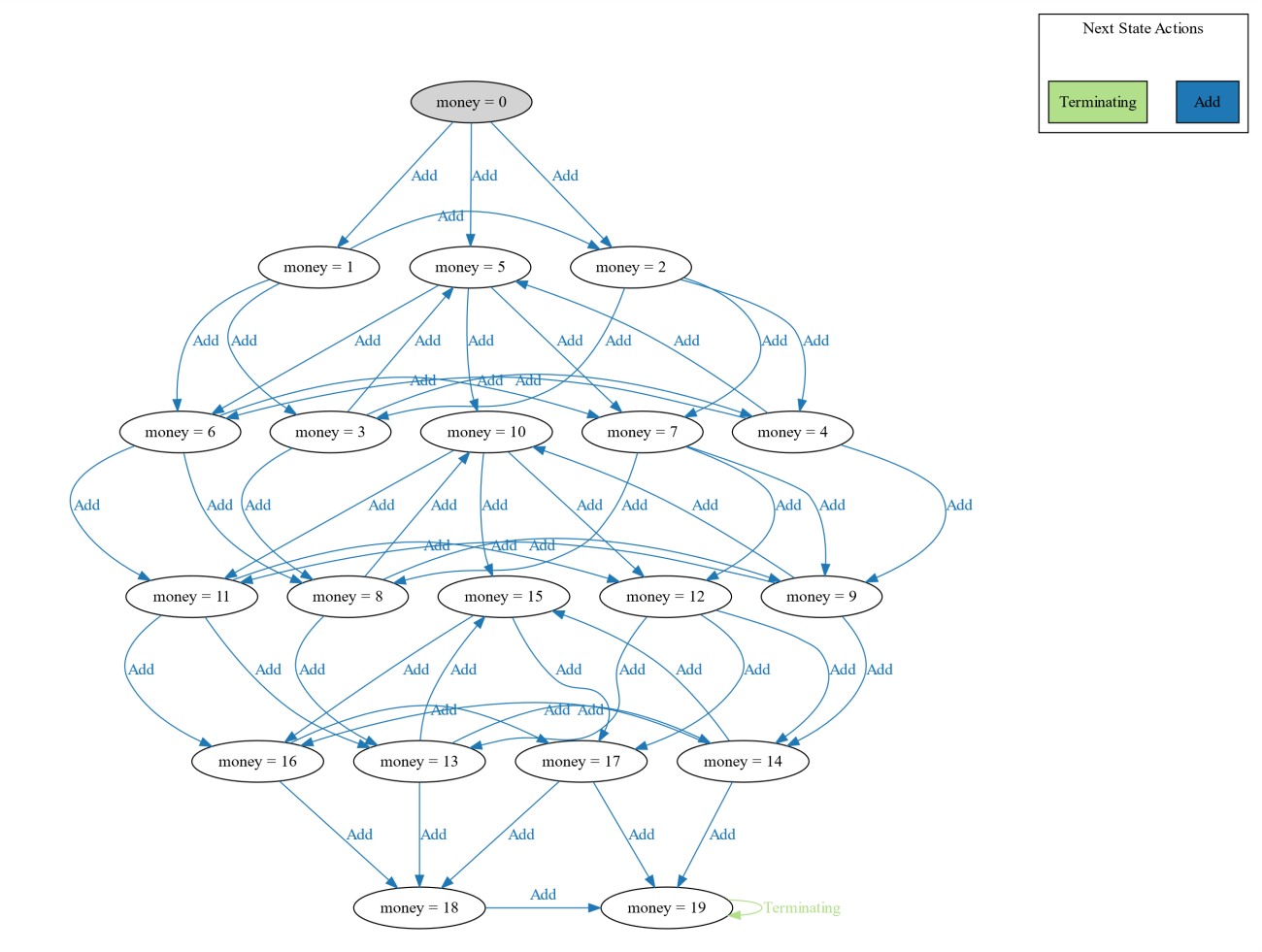Figure 8: Status Diagram

The operation of TLA+ is serial. The process of operation is to traverse the diagram on the status diagram. Each time it traverses to a status, it checks whether the current status meets the preset invariant. If it meets, it continues to traverse. If it does not meet, it will report an error immediately. TLA+ will try all traversal paths and not miss any behavior. We know that there are two ways to traverse diagrams: depth-first and breadth-first. TLA+ default breadth-first traversal can also be configured as a depth-first mode or a random behavior mode, which requires a given maximum depth.

Now, we know that the principle of TLA+ is the process of traversing and checking the status diagram. This process seems simple, but it can cover all the paths of the algorithm without missing any kind of behavior. We often use TLA+ to check the Safety and Liveness attributes of the algorithm.

## TLA+ Concurrency

You may have a preliminary understanding of the principle of TLA+, but some may still have questions in their minds. The TLA+ running process is serial, but how can the serial running TLA+ simulate concurrent algorithms or distributed algorithms?

For the serial algorithm, the action in the algorithm is Totally Ordered, which is a serial status machine, and it is easy to construct a status diagram. However, the action in the concurrent algorithm or distributed algorithm is Partially Ordered, not a serial status machine. How do you construct a status diagram?

If the actions in the concurrent algorithm or distributed algorithm can also become Totally Ordered, it can also be regarded as a serial status machine to construct a status diagram.

Lamport studied this problem early. In his most cited paper entitled Time, Clocks and the Ordering of Events in a Distributed System, he gave a method to sequence events in distributed systems. Simply put, on the premise of ensuring the order of events with a Partially Ordered relationship, the remaining unordered events are artificially ordered. All events can be ordered into Totally Ordered, and this order will not destroy causality.

TLA+ shines in the fields of concurrent algorithms and distributed algorithms because the behaviors of algorithms in these fields are diverse and prone to be neglected. Therefore, it is necessary for TLA+ to comprehensively check all the paths of the algorithm without missing any kind of behavior.

## Summary

TLA+ uses powerful computing capabilties to search for all possible behaviors of the algorithm to find unexpected behaviors. With the improvement of computing power and the increasing complexity of software and hardware systems, TLA+ will attract more attention and become an essential skill for engineers.

Finally, if you are interested in TLA+, there is a TLA+ introductory book entitled Practical TLA+. You can download the electronic edition online for free.

0 0 0
Share on